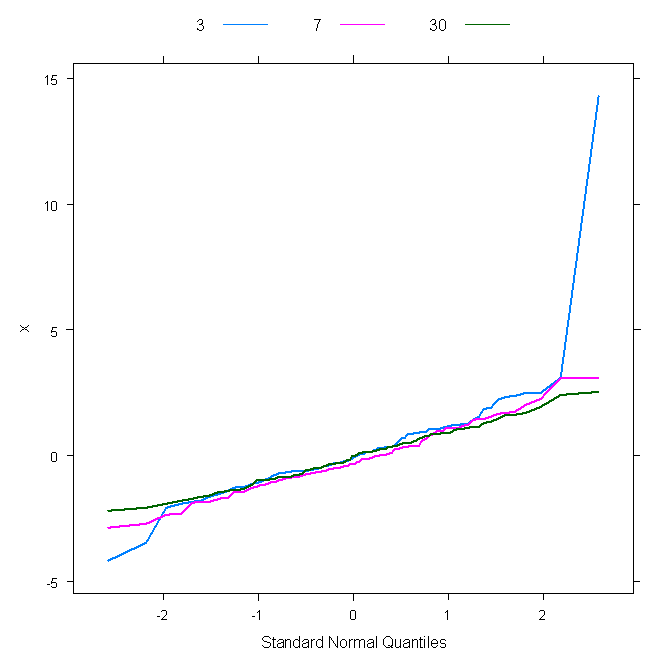Mike Livermore – CSI 773 – Week 3 Assignment – QQPlots – September 2010

X = 3.2, y = 7.4

X = 3.5, y = 11.0

X = 3.7, y = 13.4

1.3: Interpolation Plot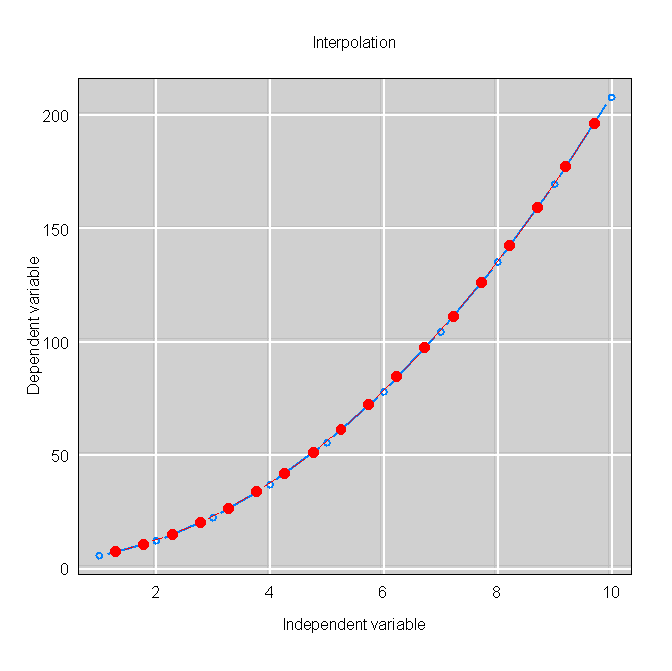2.1: QQPlot – Normal to Uniform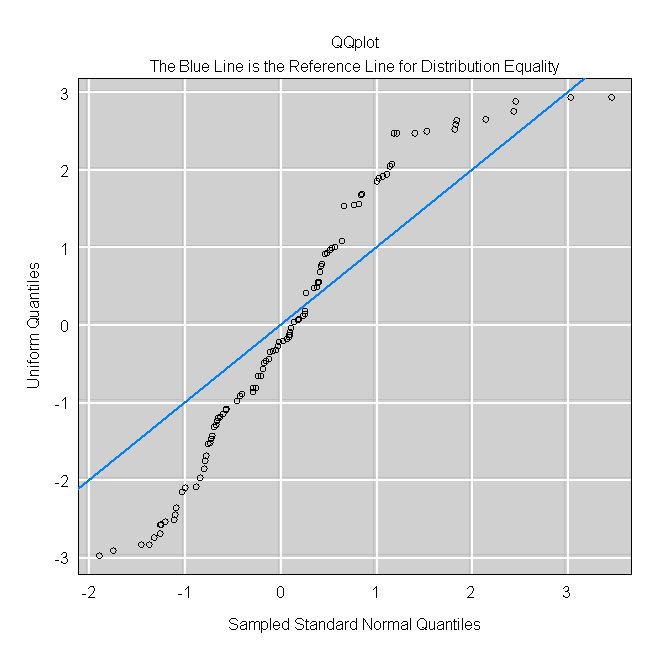2.2: QQPlot with Different Sample sizes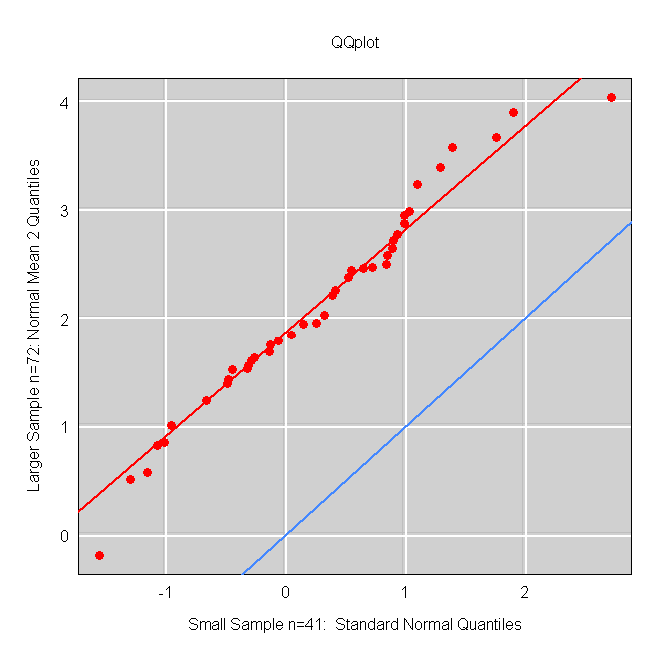2.4: My Preference

gPlot(set1Quants,set2Quants,type='n',

main="QQplot",

xlab='Sampled Standard Normal Quantiles',

ylab='Uniform Quantiles',gridLwd=1)

abline(c(0,1),lwd=2,col="blue")    # reference line for the same distribution

points(set1Quants,set2Quants,cex=1.3,pch=21,col="black",bg="red")

mtext(side=3,line=.3,"The Blue Line is the Reference Line for Distribution Equality")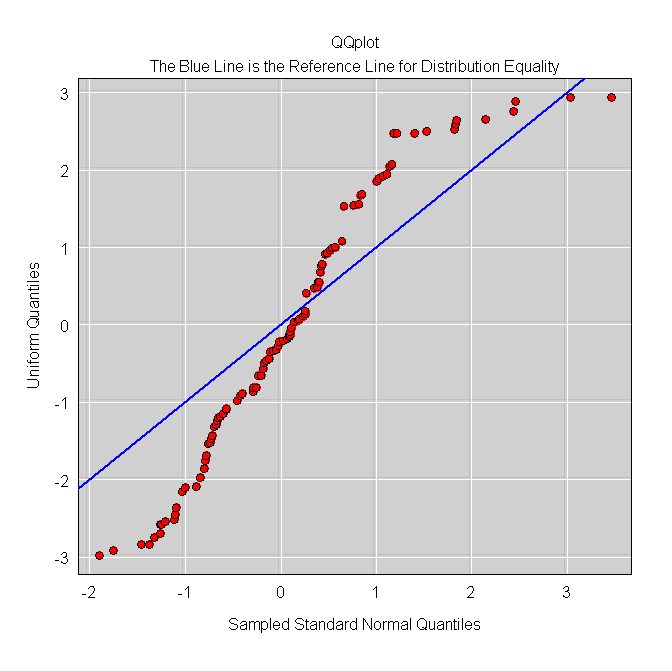3: Normal/Uniform Gaps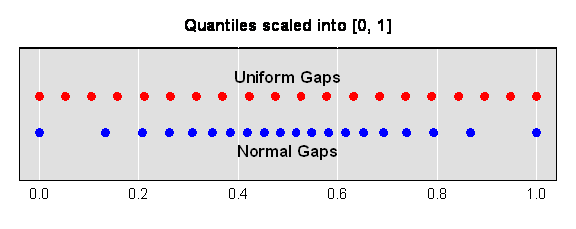4: t3 QQPlot4: Uniform QQPlot4:Weibull QQPlot5: Lattice example